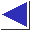# comp.lang.c FAQ list ·Question 20.31

Q: How can I find the day of the week given the date?

A: Here are three methods:

1. Use mktime or localtime (see question 13.13). Here is a code fragment which computes the day of the week for February 29, 2000:
```#include <stdio.h>
#include <time.h>

char *wday[] = {"Sunday", "Monday", "Tuesday", "Wednesday",
"Thursday", "Friday", "Saturday"};

struct tm tm;

tm.tm_mon = 2 - 1;
tm.tm_mday = 29;
tm.tm_year = 2000 - 1900;
tm.tm_hour = tm.tm_min = tm.tm_sec = 0;
tm.tm_isdst = -1;

if(mktime(&tm) != -1)
printf("%s\n", wday[tm.tm_wday]);
```
When using mktime like this, it's usually important to set tm_isdst to -1, as shown (especially if tm_hour is 0), otherwise a daylight saving time correction could push the time past midnight into another day.
2. Use Zeller's congruence, which says that if

```	J is the number of the century [i.e. the year / 100],
K the year within the century [i.e. the year % 100],
m the month,
q the day of the month,
h the day of the week [where 1 is Sunday];
```

and if January and February are taken as months 13 and 14 of the previous year [affecting both J and K]; then h for the Gregorian calendar is the remainder when the sum

```	q + 26(m + 1) / 10 + K + K/4 + J/4 - 2J
```

is divided by 7, and where all intermediate remainders are discarded. [footnote] The translation into C is straightforward:
```	h = (q + 26 * (m + 1) / 10 + K + K/4 + J/4 + 5*J) % 7;
```
(where we use +5*J instead of -2*J to make sure that both operands of the modulus operator % are positive; this bias totalling 7*J will obviously not change the final value of h, modulo 7).
3. Use this elegant code by Tomohiko Sakamoto:
```int dayofweek(int y, int m, int d)	/* 0 = Sunday */
{
static int t[] = {0, 3, 2, 5, 0, 3, 5, 1, 4, 6, 2, 4};
y -= m < 3;
return (y + y/4 - y/100 + y/400 + t[m-1] + d) % 7;
}
```